# Equivalent circuit of the waveguide application problem

• seregaxvm
In summary, the equivalent circuit method yields incorrect results when connecting two RF structures via a waveguide. The FEM solution shows that reflection should not be much higher than for the single structure.

#### seregaxvm

Hello!

I'm trying to simulate a connection between two 2-port devices via waveguide but calculated results do not agree with those, calculated in simulation software. All calculations are in the notebooks.

I took a two-port device and compared its S11 characteristic calculated using equivalent circuit with the one, calculated by FEA. Results were similar.

Then I took two similar devices, connected them via waveguide and repeated the calculation procedure. This time S11 characteristics calculated using equivalent circuit in range of waveguide lengths did not match those obtained by FEM software at all.

I checked all my math with sympy so chance of it being the equation transformation error is very low. I suspect that either there's something missing in the equivalent circuit of the waveguide or application of equivalent circuit method is incorrect here fore some reason. Can you, please, help me figure out why calculations yield wrong results?

I can’t read any of your Files so my comments are going to be generic. Microwave components connected to each other in cascade are commonly analyzed with matrix analysis. There are a number of different methods, such as ABCD matrices and T matrices. Are you using them? Are you taking into account waveguide properties such as impedance, center frequency and bandwidth, and length (phase shift)? Look in a good microwave book like Collin or Pozar for details.

Don't know, why you can't see the files. They should be available is as a preview. I'll repeat it here.

I have an RF structure: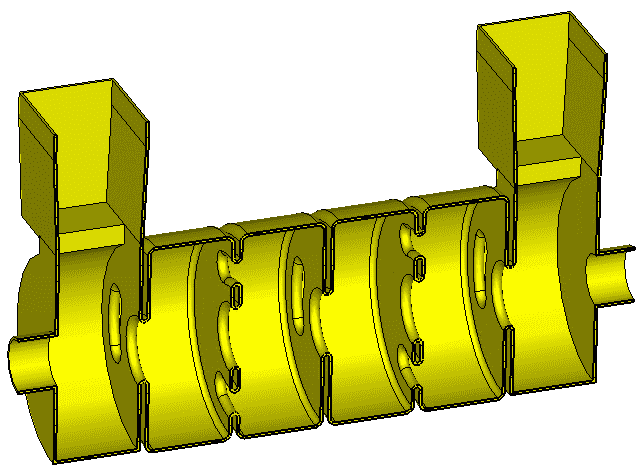which I represent as an equivalent circuit: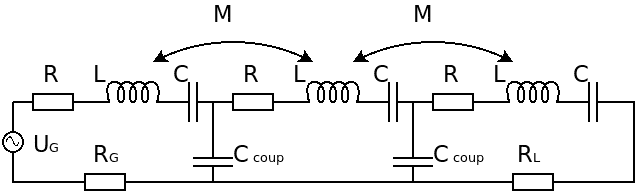and it works pretty well.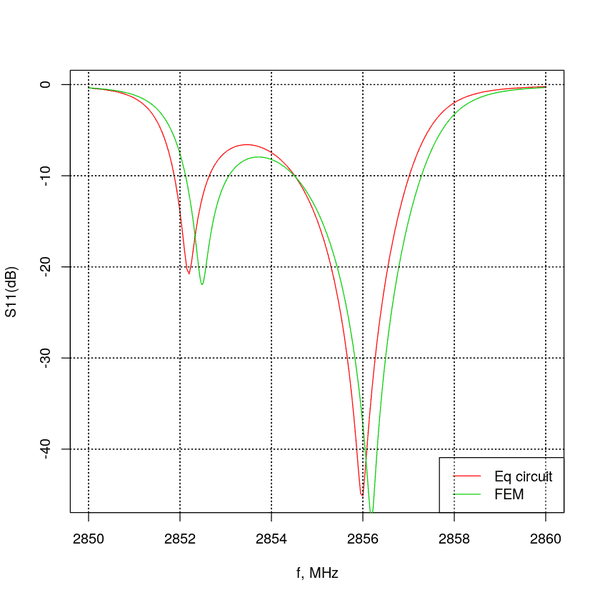Now I want to add a waveguide between them. So I take an equivalent T-circuit of the waveguide: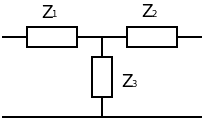$$Z_1 = Z_2 = Z_0 \tanh{\frac{l\gamma}{2}}\\ Z_3 = Z_0 \frac{1}{\sinh{l\gamma}}$$

In my case waveguide is lossless and operates above its cutoff frequency. So it should behave like an ideal phase shiftier when its length changes.
Now, to simulate the connection of two RF structures, connected by a waveguide, I take their equivalent circuits and connect them with the circuit equivalent of the waveguide: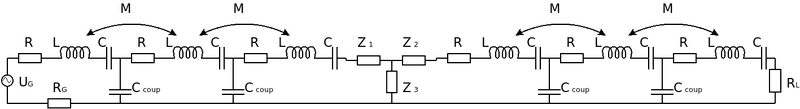The calculation result is (for different l):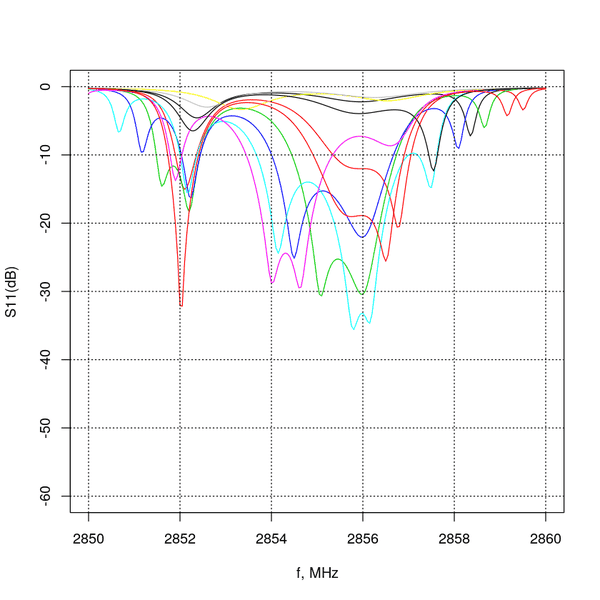While the FEM calculation is: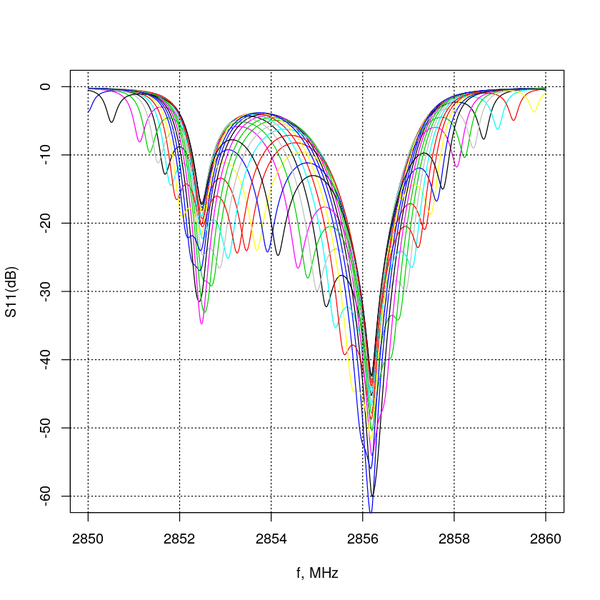In terms of incident and reflected waves, RF structures are tuned to not have a reflected wave in frequency 2856. When the length of the waveguide is changed, reflection should not be (much) higher than for the single structure. That is what FEM solution shows. Equivalent circuit solution gives a completely different result.

PS
FEM solution is setup like so: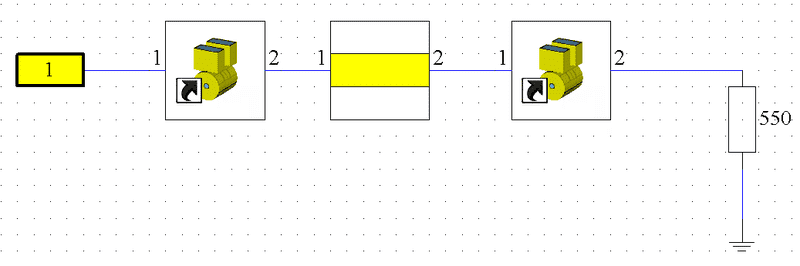S matrix of a devise is calculated numerically (it matches the result from the equivalent circuit). All other calculations are done using these S matrices and S matrices of an ideal transmission line and load with matching impedance.
In my mind operations in terms of S matrices and Z matrices should yield the same results but they don't.
PPS
I checked equation transformations with symbolic math library sympy so the error in them is unlikely.

#### Attachments

I notice you have some loss in the networks. This will cause Zo to be complex I think. Have you picked this up in the two analyses?

Actually, loss is characterized by a real part of the Z. Imaginary part represents reactive elements of the circuit. Yes, I do all my calculations with complex numbers.

seregaxvm said:
Actually, loss is characterized by a real part of the Z. Imaginary part represents reactive elements of the circuit. Yes, I do all my calculations with complex numbers.
But I notice that you terminate the networks with 550R. As there is loss in the networks, I expect Zo to have a reactive component.

The reason for this is that normally device operates on the resonant frequency. On the resonance the reactive component of the impedance is zero. So, this kind of devices is always terminated with a restive load.

seregaxvm said:
The reason for this is that normally device operates on the resonant frequency. On the resonance the reactive component of the impedance is zero. So, this kind of devices is always terminated with a restive load.
Notice a distinction between the input impedance of the line and the characteristic impedance of the line. I am suggesting that Zo is reactive. The line will be properly terminated when it is provided with a load with the magnitude of Zo and the opposite sign. So for a lossy line, zero reflection will require a reactive load.
These effects are very small, but you are looking for very small errors in your plots.

Not sure what are you talking about. I'm looking for huge errors. The first plot, with two lines on it, is an example of a good matching results. I'm comparing two last plots between them (each calculated for different length of waveguide).